Help Center

# Checker file

## Programming languages supported

In a test, the checker file is supported in the following languages only:

1. Python
2. Python 3
3. Python 3.8
4. R (RScript)

Note: HackerEarth uses Python 3 to create checker files. Thus, the screenshot of codes provided in the following sections are in Python 3.

## Structure of a checker file

A checker file contains the following two functions:

• verify_submission: It verifies a candidate’s submission file based on certain checkpoints. Some of these checkpoints include checking the file type, number of rows present, number of columns present, predictions for all IDs provided in the test case, and so on.
• gen_score: It generates a score for the candidate’s submission based on an evaluation metric.

Notes

• The test case file represents the result file or truth values.
• The submission file represents a candidate’s submission file.

The different segments in a checker file are as follows:

1. Importing libraries
You have to import all the required libraries. This is shown in the following image: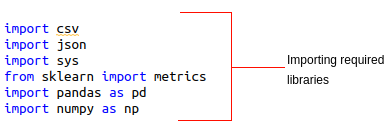The following code allows you to  load the test case and submission file: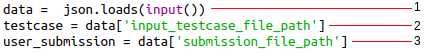The description of the labels are as follows:

1. A JSON file is initialized to the data variable. This file contains the path of the test case and user submission.
2. The testcase variable is assigned the path for the test case file.
3. The user_submission variable is assigned the path of the submission file.
1. The execution starts  with the verify_submission function (labelled as 1). If an exception is raised based on the checkpoints provided in the verify_submission function, it is displayed to the candidate (labelled as 2). This is performed by using the following code: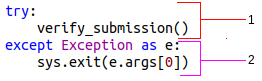2. The verify_submission() function
You initialize the following two variables:
• message: Represents an empty list that is used to append messages if a certain checkpoint fails
• score: Represents a hard coded value (equal to 0) that is displayed if a submission fails a checkpoint
1. The ID variable represents the name of the index column. You are required to replace None with the name of the index column.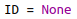Example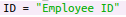1. Checkpoint 1: The platform supports .csv, .txt, and .json types of submission files. You can assign one of these file extensions to the file_ending_with variable as shown in the following code: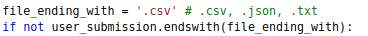If the file is not in the specified format, then the following message is appended to the
message list:

1. The fp_submission variable (labelled as 1) reads the candidate's submission and the fp_testcase variable (labelled as 2) reads the test cases of a  question.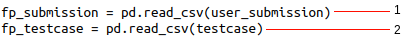1. Checkpoint 2:The following code assigns the number of rows in a test case to the num_rows variable.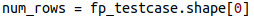The following code checks whether the number of rows of a submission is equal to the number of rows of a test case: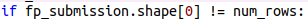Note: This step is dynamic.

• When you click Compile & Test, the num_rows variable is assigned the length of the sample test data or sample expected result.
• When you click Submit, the num_rows variable is assigned the length of the complete test data or complete expected result.
• If the numbers of rows are not equal, then the following message is appended to the message list:
1. Checkpoint 3: The candidate's submission must contain the following:
• All the columns that are provided in the test case file.
• All column names must be written in the same format as mentioned in the sample submission file.

To check whether the columns in test case is same as submission, you use the following code: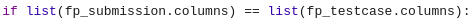In any case, if the submission file does not contain a column that is provided in the test case, then the following message is appended to the
message list:

1. The following two lines of code sets ID as the index value for the test case (labelled as 1) and candidate’s submission (labelled as 2):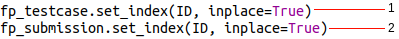1. The label_cols variable extracts the names of the columns that are available in the test case.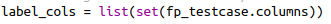1. An intersection of all the index values that are present in the test case and candidate's submission are stored in the intersection variable.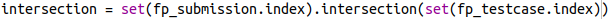2. Checkpoint 4: Sometimes, a candidate can miss certain index values. The set difference between the test case index values and the submission index values  must be equal to zero. This is performed by using the following code: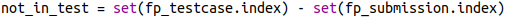Here, the
not_in_test variable stores the indexes that are missing in the candidate’s submission.
Further, if the length of
not_in_test is equal to 0, that is, there are no missing index values and the submission is passed for further evaluations..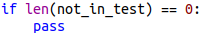If there are missing index values, then these values are stored in the
key_not_found variable.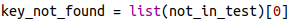All the missing index values are added to the following error message that is appended to the message list:

1. The index values that are stored in the intersection variable are used to pick the prediction values from the test case (labelled as 1) and candidate’s submission (labelled as 2).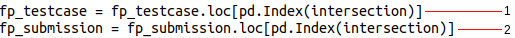2. All the required columns that are stored in label_cols (mentioned in point no. 11) are extracted from the test case and submission. These columns along with their respective prediction values are initialized in the actual_values (labelled as 1) and predicted_values (labelled as 2) variables, respectively.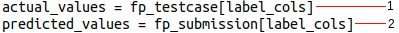Then, the actual values and predicted values are passed in the
gen_score function as shown in  the following code: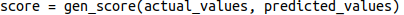1. The gen_score() function

This function takes two arguments

• actual parameter: Represents the truth values of the target column(s) in the test case
• predicted parameter: Represents the predictions of the target column(s) in a candidate's submission.

Here, the score variable contains a formula based on an evaluation metric.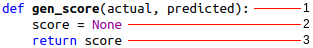The description of the labels are as follows:

• The gen_score function with two parameters as mentioned.
• A score is calculated based on an evaluation metric and it is assigned to the score variable.
• The function returns the value of score after the required calculation in the previous step.

Example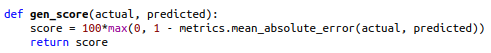1. Final scoring
If the length of the message list is zero, then you can print the score achieved (from gen_score) and a message called ‘Successful’ to represent successful compilation and submission of the candidate's submission.

Otherwise, display score = 0 and the message that represents a hint about an incorrect submission. The code for this is as follows: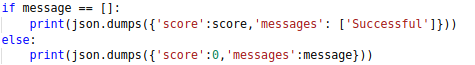An example of the execution of checker file from the candidate interface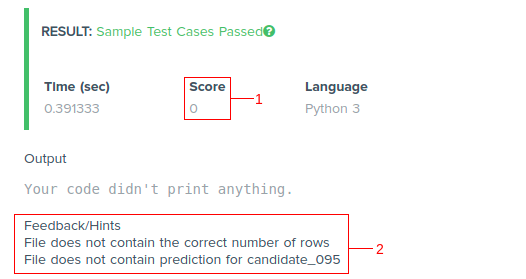The Score is equal to zero (labelled as 1) because the submission failed at two checkpoints that are displayed in the Feedback/Hints section (labelled as 2).

Once you have understood the structure of a checker file, you can easily create your own. You can refer to the sample checker file.